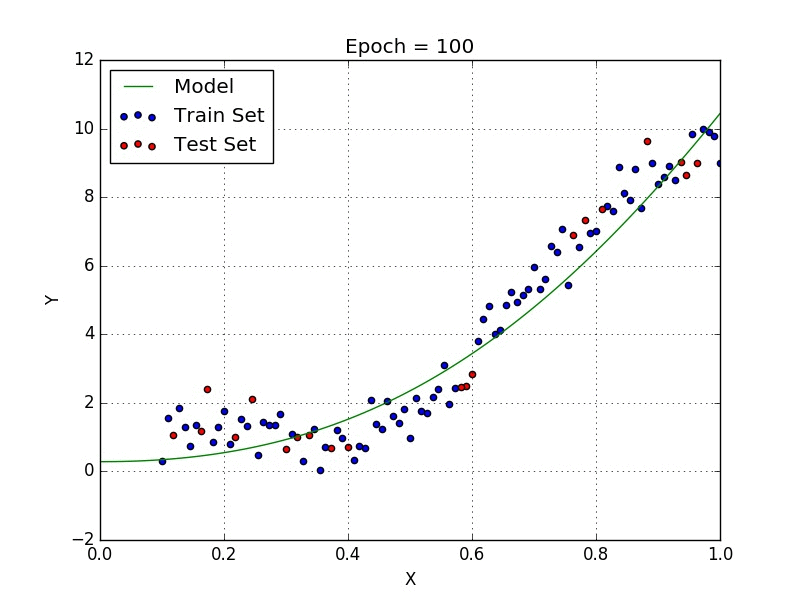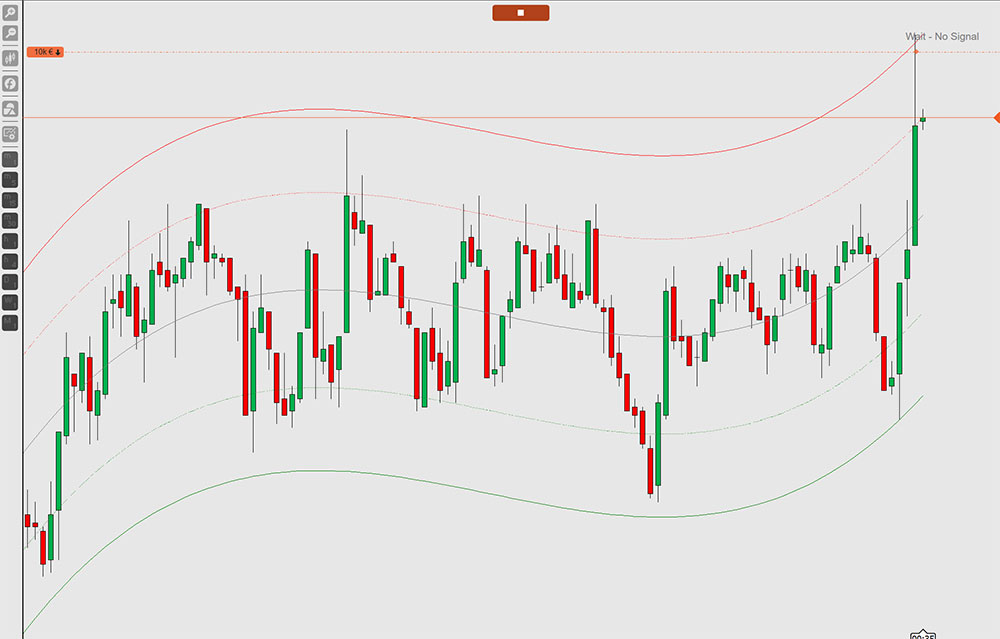# How to Trade Using Polynomial Regression Channels

If you want to find out more about Polynomial Regression Channels and how you can trade with them either manually or automated then this is a good place to start.

## What Is a Polynomial Regression (PRC) Indicator?

The word PRC stands for Polynomial Regression Channel. This is a regression indicator that is used on charts. A linear regression indicator draws a straight line of best fit on a chart. The PRC indicator applies the polynomial function to the linear regression functions to adapt itself to market flow.

## How Does Polynomial Regression Work?

In statistics, polynomial regression is a form of regression analysis in which the relationship between the independent variable x and the dependent variable y is modelled as an nth degree polynomial in x. Simply put polynomial regression is an attempt to create a polynomial function that approximates a set of data points. With polynomial regression, the data is approximated using a polynomial function. A polynomial is a function that takes the form fx ) = c0 + cx + c2 x2 ⋯ cn xn where n is the degree of the polynomial and c is a set of coefficients.If we try to use a simple linear regression in the above graph then the linear regression line will not fit very well. It is very difficult to fit a linear regression line with a low error value, so we can try to use the polynomial regression formula to fit a polynomial line so that we can achieve a minimum cost function.

## How to use Polynomial Regression for Trading?

A common indicator that is used by many trading platforms is called the linear or polynomial regression channel, this creates boundaries around the price action where the price found support and resistance from the bulls and the bears, the image below shows this in action. You can see the PRC formula has 5 lines, at the top and the bottom, you have the outer and inner band of the channel and in the centre, you have the middle band which is deemed as neutral.